# 1 Introduction

`randRotation` is an R package intended for generation of randomly rotated data to resample null distributions of linear model based dependent test statistics. See also (Yekutieli and Benjamini 1999) for resampling dependent test statistics. The main application is to resample test statistics on linear model coefficients following arbitrary batch effect correction methods, see also section Quick start. The random rotation methodology is thereby applicable for linear models in combination with normally distributed data. Note that the resampling procedure is actually based on random orthogonal matrices, which is a broader class than random rotation matrices. Nevertheless, we adhere to the naming convention of (Langsrud 2005) designating this approach as random rotation methodology. Possible applications for resampling by roation, that are outlined in this document, are: (i) linear models in combination with practically arbitrary (linear or non-linear) batch effect correction methods, section 6; (ii) generation of resampled datasets for evaluation of data analysis pipelines, section 6.2; (iii) calculation of resampling based test statistics for calculating resampling based p-values and false discovery rates (FDRs), sections 6.2 and 6.3; and (iv) estimation of the degrees of freedoms (df) of mapping functions, section 8.

Generally, the rotation approach provides a methodology for generating resampled data in the context of linear models and thus potentially has further conceivable areas of applications in high-dimensional data analysis with dependent variables. Nevertheless, we focus this document on the outlined range of issues in order to provide an intuitive and problem-centered introduction.

# 2 Installation

Execute the following code to install package `randRotation`:

``````if(!requireNamespace("BiocManager", quietly = TRUE))
install.packages("BiocManager")
BiocManager::install("randRotation")``````

# 3 Sample dataset

For subsequent analyses we create a hypothetical dataset with 3 batches, each containing 5 Control and 5 Cancer samples with 1000 features (genes). Note that the created dataset is pure noise and no artificial covariate effects are introduced. We thus expect uniformly distributed p-values for linear model coefficients.

``````library(randRotation)
set.seed(0)
# Dataframe of phenotype data (sample information)
pdata <- data.frame(batch = as.factor(rep(1:3, c(10,10,10))),
phenotype = rep(c("Control", "Cancer"), c(5,5)))
features <- 1000

# Matrix with random gene expression data
edata <- matrix(rnorm(features * nrow(pdata)), features)
rownames(edata) <- paste("feature", 1:nrow(edata))

xtabs(data = pdata)
#>      phenotype
#> batch Cancer Control
#>     1      5       5
#>     2      5       5
#>     3      5       5``````

# 4 Quick start - linear models with batch effect correction

A main application of the package is to resample null distributions of parameter estimates for linear models following batch effect correction. We first create our model matrix:

``````mod1 <- model.matrix(~1+phenotype, pdata)
#>   (Intercept) phenotypeControl
#> 1           1                1
#> 2           1                1
#> 3           1                1
#> 4           1                1
#> 5           1                1
#> 6           1                0``````

We then initialise the random rotation object with `initBatchRandrot` and select the `phenotype` coefficient as the null hypothesis coefficient:

``````rr <- initBatchRandrot(Y = edata, X = mod1, coef.h = 2, batch = pdata\$batch)
#> Initialising batch "1"
#> Initialising batch "2"
#> Initialising batch "3"``````

Now we define the data analysis pipeline that should be run on the original dataset and on the rotated dataset. Here we include as first step (I) our batch effect correction routine `ComBat` (sva package) and as second step (II) we obtain the coefficient of interest from the linear model fit.

``````statistic <- function(Y, batch, mod){
# (I) Batch effect correction with "Combat" from the "sva" package
Y <- sva::ComBat(dat = Y, batch = batch, mod = mod)

# (II) Linear model fit
fit1 <- lm.fit(x = mod, y = t(Y))
abs(t(coef(fit1))[,2])
}``````

Note that larger values of the `statistic` function are considered as more significant in the subsequently used `pFdr` function. We thus take the absolute values of the coefficients in order to calculate two-sided (two-tailed) p-values with `pFdr`. We emphasize that we highly recommend using scale independent statistics (pivotal quantities) as e.g. t-values instead of parameter estimates (as with `coef`), see also `?randRotation::pFdr`. Nevertheless, we use `coef` here in order to avoid bulky function definitions.

The `rotateStat` function calculates `statistic` on the original (non-rotated) dataset and on 10 random rotations. `batch` and `mod` are provided as additional parameters to `statistic`.

``````rs1 <- rotateStat(initialised.obj = rr, R = 10, statistic = statistic,
batch = pdata\$batch, mod = mod1)``````
``````rs1
#> Rotate stat object
#>
#> R = 10
#>
#> dim(s0): 1000 1
#>
#> Statistic:
#> function(Y, batch, mod){
#>   # (I) Batch effect correction with "Combat" from the "sva" package
#>   Y <- sva::ComBat(dat = Y, batch = batch, mod = mod)
#>
#>   # (II) Linear model fit
#>   fit1 <- lm.fit(x = mod, y = t(Y))
#>   abs(t(coef(fit1))[,2])
#> }
#> <bytecode: 0x562a44e008b0>
#>
#> Call:
#> rotateStat(initialised.obj = rr, R = 10, statistic = statistic,
#>     batch = pdata\$batch, mod = mod1)``````

Resampling based p-values are obtained with `pFdr`. As we use “pooling” of the rotated statistics in `pFdr`, 10 random rotations are sufficient.

``````p.vals <- pFdr(rs1)
hist(p.vals, col = "lightgreen");abline(h = 100, col = "blue", lty = 2)``````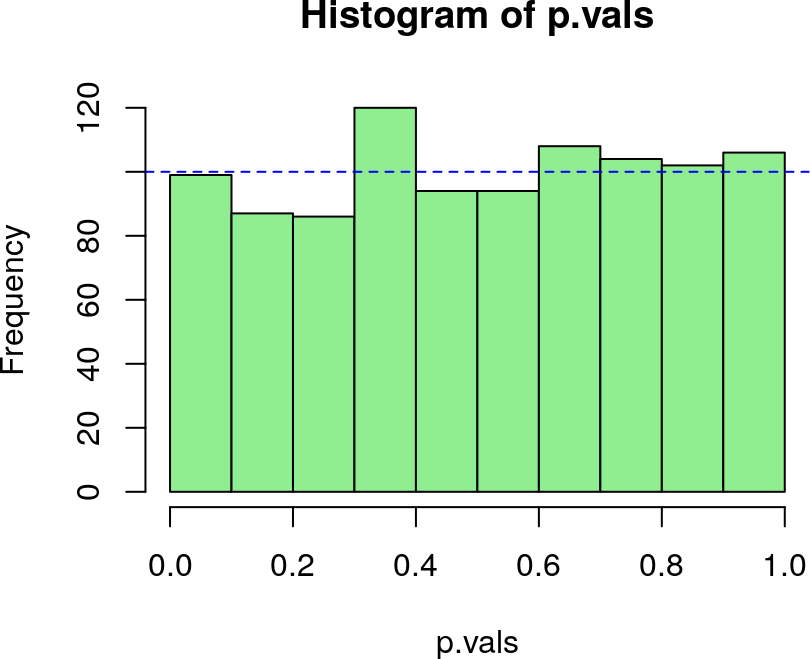``qqunif(p.vals)``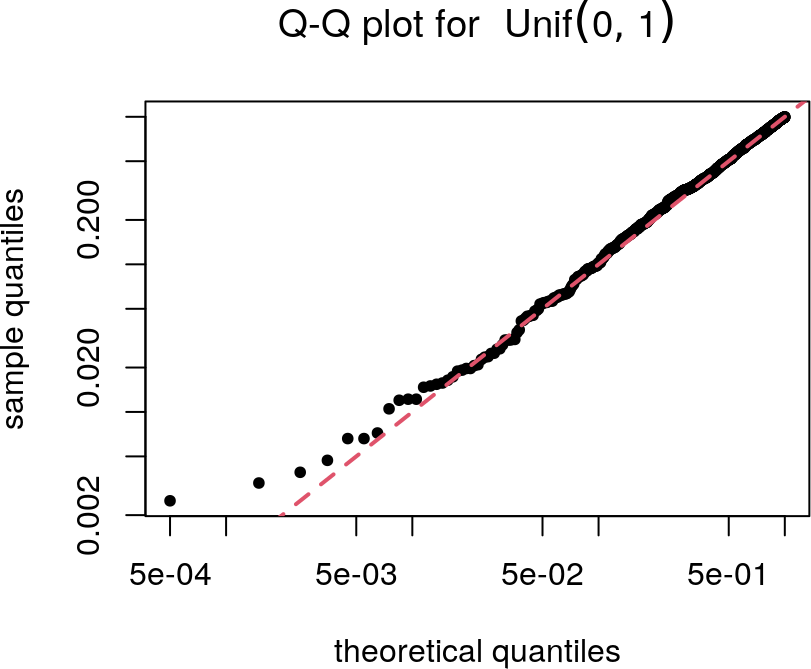We see that, as expected, our p-values are approximately uniformly distributed.

Hint: The outlined procedure also works with `statistic` functions which return multiple columns (`rotateStat` and `pFdr` handle functions returning multiple columns adequately). So one could e.g. perform multiple batch effect correction methods and calculate the statistics of interest for each correction method. By doing this, one could subsequently evaluate the influence of different batch effect correction methods on the statistic of interest.

Additional info: Below, the analysis pipeline is performed without rotation for comparison with the previous analyses. Following batch effect correction with `ComBat` (sva package), we obtain p-values from linear fit coefficients as follows:

``````edata.combat <- sva::ComBat(dat = edata, batch = pdata\$batch, mod = mod1)
#> Found3batches
#> Adjusting for1covariate(s) or covariate level(s)
#> Standardizing Data across genes
#> Fitting L/S model and finding priors
fit1 <- lm.fit(x = mod1, y = t(edata.combat))

# t-statistics
var.beta <- diag(solve(t(mod1)%*%mod1))
s2 <- colSums(resid(fit1)^2) / df.residual(fit1)
t1 <- t(coef(fit1) / sqrt(var.beta)) / sqrt(s2)

# P-values from t-statistics
p.vals.nonrot <- 2*pt(abs(t1), df.residual(fit1), lower.tail = FALSE)
p.vals.nonrot <- p.vals.nonrot[,2]

hist(p.vals.nonrot, col = "lightgreen");abline(h = 100, col = "blue", lty = 2)``````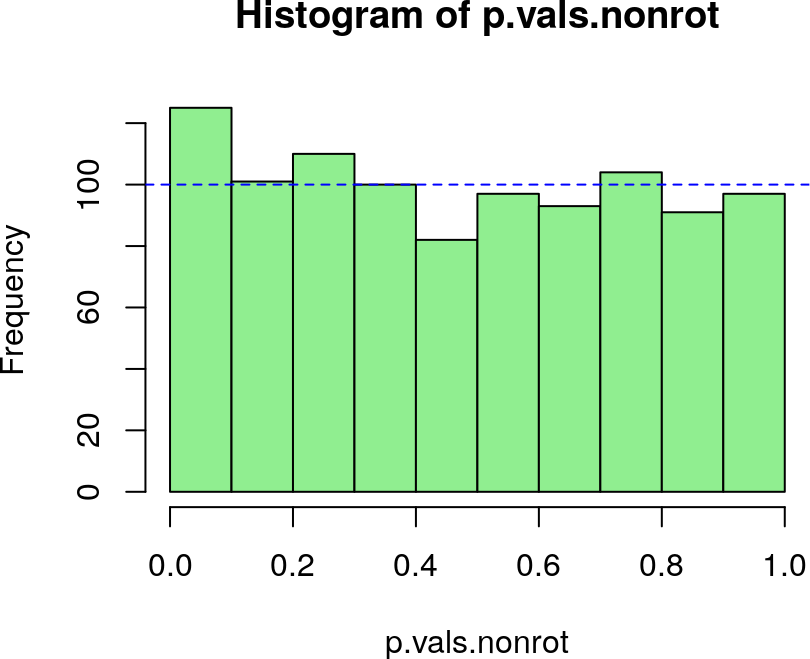``qqunif(p.vals.nonrot)``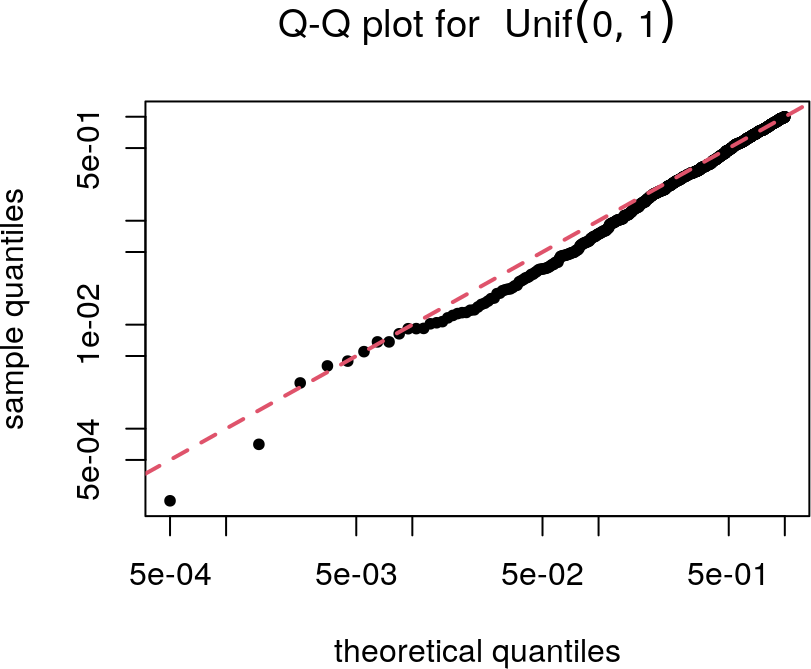``````plot(p.vals, p.vals.nonrot, log = "xy", pch = 20)
abline(0,1, col = 4, lwd = 2)``````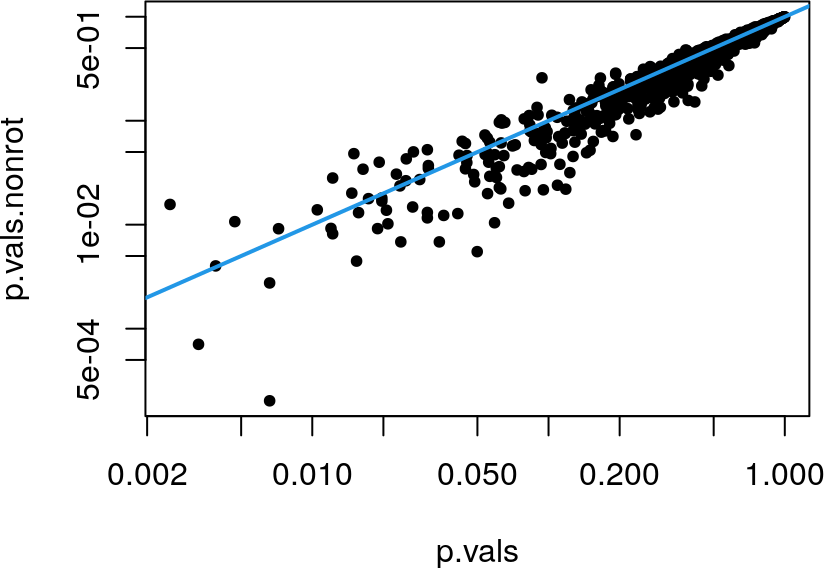We see that the p-values are non-uniformly distributed. See also section 6.1.

# 5 Basic principle of random rotation methods

In the random rotation methodology, the observed data vectors (for each feature) are rotated in way that the determined coefficients (\(\boldsymbol{B_D}\) in Langsrud (2005)) stay constant when resampling under the null hypothesis \(H0: \boldsymbol{B_H = 0}\), see (Langsrud 2005).

The following example shows that the intercept coefficient of the null model does not change when rotation is performed under the null hypothesis:

``````# Specification of the full model
mod1 <- model.matrix(~1+phenotype, pdata)

# We select "phenotype" as the coefficient associated with H0
# All other coefficients are considered as "determined" coefficients
rr <- initRandrot(Y = edata, X = mod1, coef.h = 2)

coefs <- function(Y, mod){
t(coef(lm.fit(x = mod, y = t(Y))))
}

# Specification of the H0 model
mod0 <- model.matrix(~1, pdata)

coef01 <- coefs(edata, mod0)
coef02 <- coefs(randrot(rr), mod0)

#>            (Intercept)  (Intercept)
#> feature 1  0.040776840  0.040776840
#> feature 2 -0.001668893 -0.001668893
#> feature 3  0.036254408  0.036254408
#> feature 4 -0.272031781 -0.272031781
#> feature 5  0.105838531  0.105838531
#> feature 6 -0.012137419 -0.012137419

all.equal(coef01, coef02)
#>  TRUE``````

However, the coefficients of the full model do change when rotation is performed under the null hypothesis:

``````coef11 <- coefs(edata, mod1)
coef12 <- coefs(randrot(rr), mod1)

#>            (Intercept) phenotypeControl (Intercept) phenotypeControl
#> feature 1  0.236257608      -0.39096154 -0.30039880       0.68235129
#> feature 2  0.023970184      -0.05127815  0.15804314      -0.31942406
#> feature 3  0.180283050      -0.28805729 -0.05785820       0.18822522
#> feature 4 -0.007109299      -0.52984496 -0.25565703      -0.03274951
#> feature 5  0.452219329      -0.69276160  0.09967972       0.01231761
#> feature 6  0.031196538      -0.08666791 -0.12934853       0.23442223``````

This is in principle how resampling based tests are constructed.

# 6 Batch effect correction with subsequent linear model analysis

In the following we outline the use of the `randRotation` package for linear model analysis following batch effect correction as a prototype application in current biomedical research. We highlight the problems faced when batch effect correction is separated from data analysis with linear models. Although data analysis procedures with combined batch effect correction and model inference should be preferred, the separation of batch effect correction from subsequent analysis is unavoidable for certain applications. In the following we use `ComBat` (sva package) as a model of a “black box” batch effect correction procedure. Subsequent linear model analysis is done with the limma package. We use `limma` and `ComBat` as model functions for demonstration, as these are frequently used in biomedical research. We want to emphasize that neither the described issues are specific to these functions, nor do we want to somehow defame these highly useful packages.

## 6.1 Skewed null distribution of p values

Separating a (possibly non-linear) batch effect correction method from linear model analysis could practically lead to non-uniform (skewed) null distributions of p-values for testing linear model coefficients. The intuitive reason for this skew is that the batch effect correction method combines information of all samples to remove the batch effects. After removing the batch effects, the samples are thus no longer independent. For further information please refer to section df estimation and to the references.

The following example demonstrates the influence of the batch effect correction on the distribution of p-values. We first load the limma package and create the model matrix with the intercept term and the phenotype term.

``````library(limma)

mod1 = model.matrix(~phenotype, pdata)``````

Remember that our sample dataset is pure noise. Thus, without batch effect correction, fitting a linear model with `limma` and testing the phenotype coefficient results in uniformly distributed p-values:

``````# Linear model fit
fit0 <- lmFit(edata, mod1)
fit0 <- eBayes(fit0)

# P values for phenotype coefficient
p0 <- topTable(fit0, coef = 2, number = Inf, adjust.method = "none",
sort.by = "none")\$P.Value
hist(p0, freq = FALSE, col = "lightgreen", breaks = seq(0,1,0.1))
abline(1,0, col = "blue", lty = 2)``````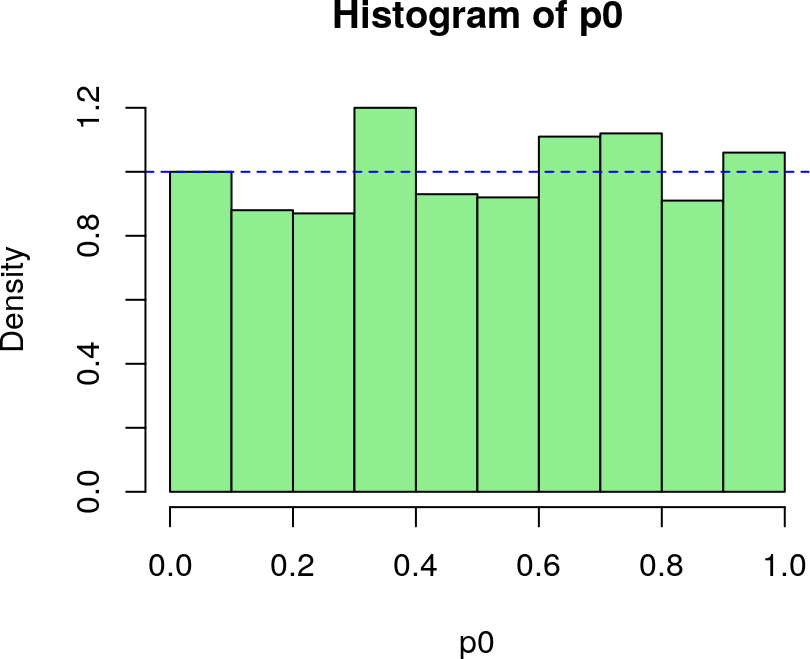``qqunif(p0)``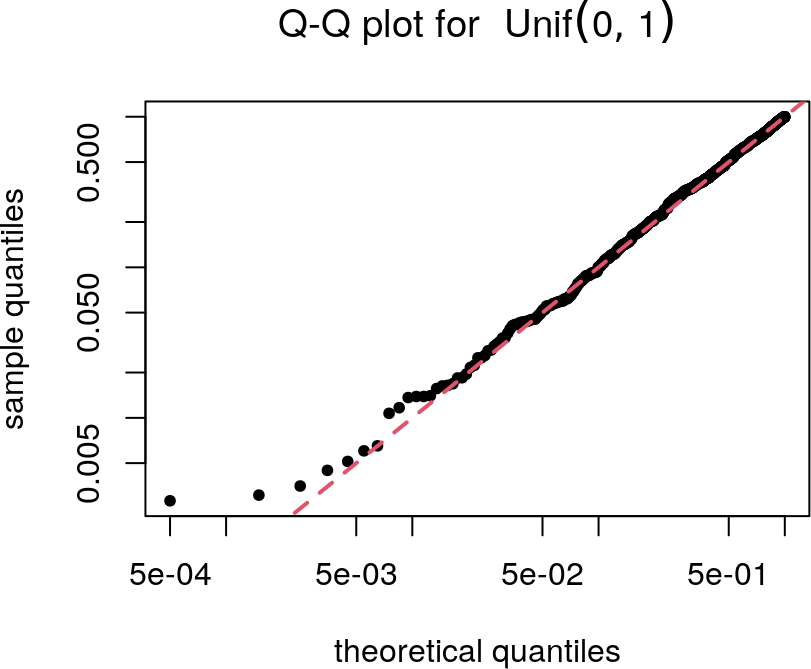We now perform batch effect correction using `ComBat` (sva package):

``````library(sva)
#> This is mgcv 1.8-31. For overview type 'help("mgcv-package")'.
edata.combat = ComBat(edata, batch = pdata\$batch, mod = mod1)
#> Found3batches
#> Adjusting for1covariate(s) or covariate level(s)
#> Standardizing Data across genes
#> Fitting L/S model and finding priors

Performing the model fit and testing the phenotype effect on this modified dataset results in a skewed p-value distribution:

``````# Linear model fit
fit1 <- lmFit(edata.combat, mod1)
fit1 <- eBayes(fit1)

# P value for phenotype coefficient
p.combat <- topTable(fit1, coef = 2, number = Inf, adjust.method = "none",
sort.by = "none")\$P.Value
hist(p.combat, freq = FALSE, col = "lightgreen", breaks = seq(0,1,0.1))
abline(1,0, col = "blue", lty = 2)``````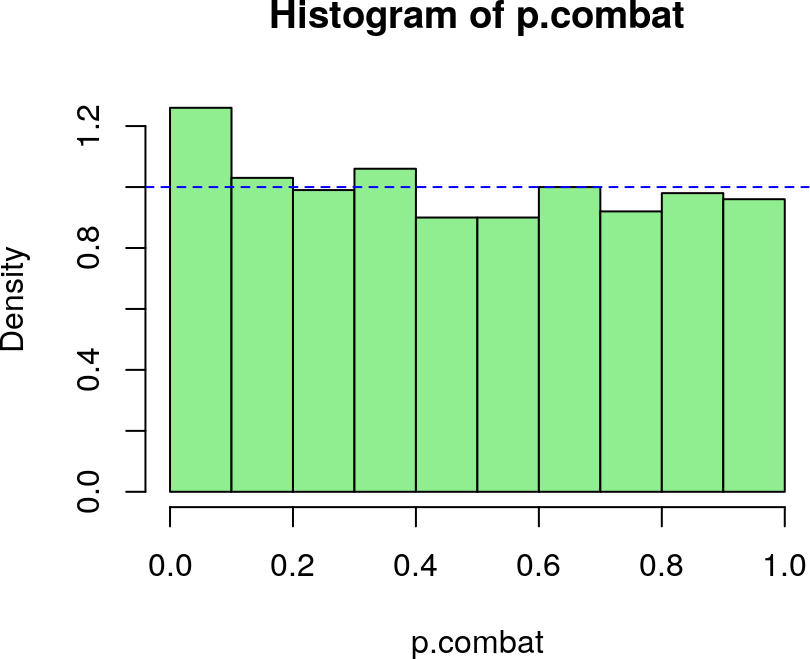``qqunif(p.combat)``